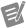Pattern Generator (Sine) (G Dataflow)

Generates a signal containing a sine pattern.amplitude

Amplitude of the pattern.

Default: 1cycles

Number of complete periods of the sine pattern.Note

Because this input is a floating-point number, fractional cycles are possible for the sine pattern. Furthermore, setting this input to a negative number does not generate an error condition because it is mathematically correct and useful to consider negative frequencies in Fourier and spectral analysis.

Default: 1phase

Phase, in degrees, of the sine wave without reset. This input must be in degrees rather than radians. If this input is in radians, convert the value to degrees before using this node.

Default: 0error in

Error conditions that occur before this node runs.

The node responds to this input according to standard error behavior.

Standard Error Behavior

Many nodes provide an error in input and an error out output so that the node can respond to and communicate errors that occur while code is running. The value of error in specifies whether an error occurred before the node runs. Most nodes respond to values of error in in a standard, predictable way.

error in does not contain an error error in contains an errorIf no error occurred before the node runs, the node begins execution normally.

If no error occurs while the node runs, it returns no error. If an error does occur while the node runs, it returns that error information as error out.

If an error occurred before the node runs, the node does not execute. Instead, it returns the error in value as error out.

Default: No errordt

Sampling rate in samples per second.

This input is available only if you configure this node to return a waveform.

Default: 0.1samples

Number of samples in the pattern. This input must be greater than or equal to 0. If this input is less than zero, the node sets the output pattern to an empty array and returns an error.

Default: 128t0

Timestamp of the output signal. If this input is unwired, this node uses the current time as the timestamp of the output signal.

This input is available only if you configure this node to return a waveform.sine pattern

Output sine pattern. The largest output pattern this node can generate depends on the amount of memory in your system and is theoretically limited to 2,147,483,647 (231 - 1) elements.

This output can return a waveform or an array of double-precision, floating-point numbers.error out

Error information.

The node produces this output according to standard error behavior.

Standard Error Behavior

Many nodes provide an error in input and an error out output so that the node can respond to and communicate errors that occur while code is running. The value of error in specifies whether an error occurred before the node runs. Most nodes respond to values of error in in a standard, predictable way.

error in does not contain an error error in contains an errorIf no error occurred before the node runs, the node begins execution normally.

If no error occurs while the node runs, it returns no error. If an error does occur while the node runs, it returns that error information as error out.

If an error occurred before the node runs, the node does not execute. Instead, it returns the error in value as error out.

Where This Node Can Run:

Desktop OS: Windows

FPGA: This product does not support FPGA devices